# 1. The pdf of Y is f(y) = k(y^3) I[2,3](y) where k is a constant, real...

1. The pdf of Y is f(y) = k(y^3) I[2,3](y) where k is a constant, real number.

(a) (5 points) Calculate the value of k so that f(y) is a valid pdf.

(b) (5 points) Find E(Y ). Hint: write k as a fraction so you don’t have to round until the end.

(c) (5 points) Find the 50th percentile of Y .

(d) (2 points) Briefly (1-2 sentences) explain why your answers to parts (b) and (c) make sense.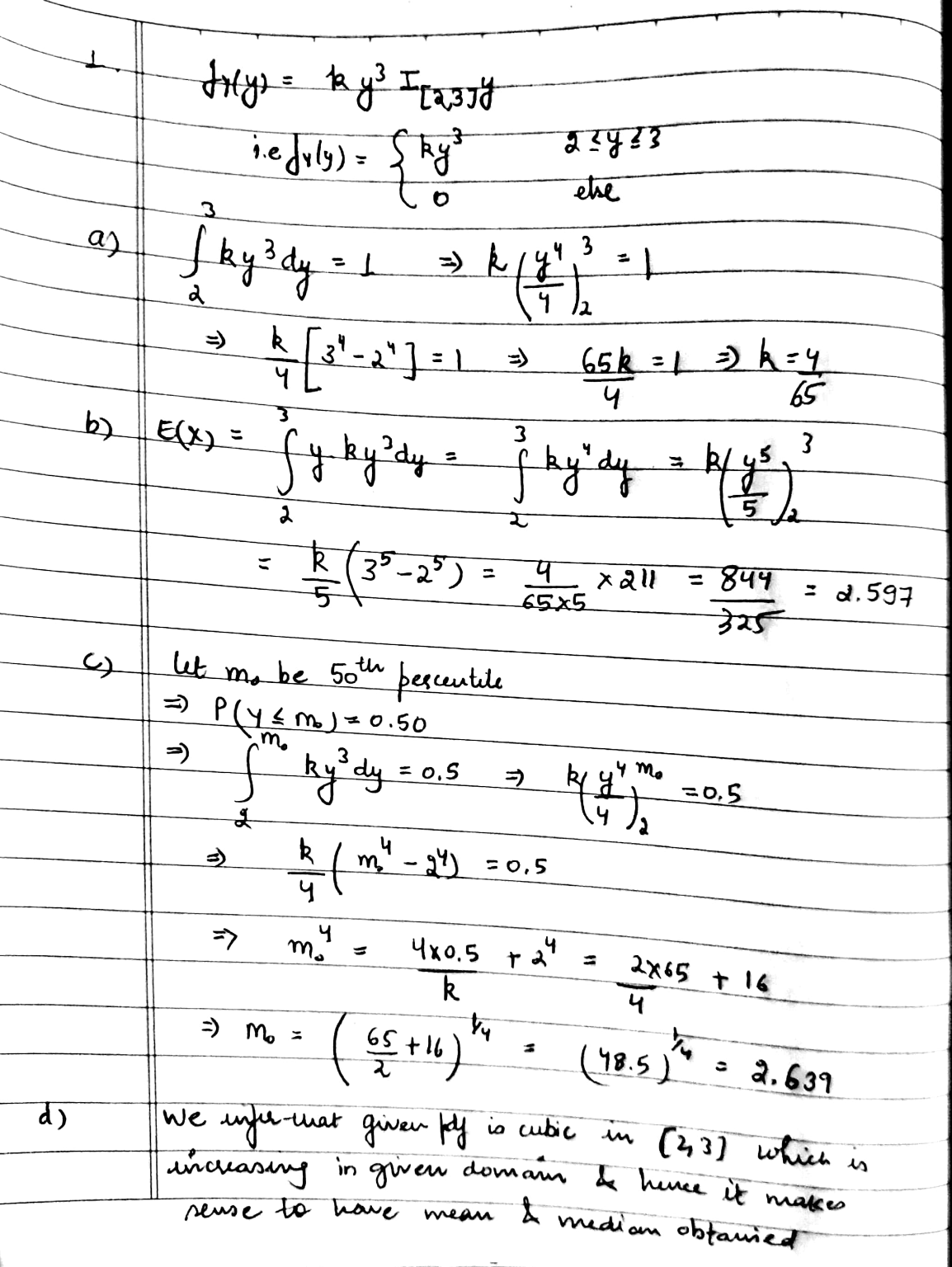#### Earn Coin

Coins can be redeemed for fabulous gifts.

Similar Homework Help Questions
• ### 0 〈 y 〈 x2く1· Consider two rvs X and Y with joint pdf f(x,y) = k-y, (a) Sketch the region in two dimensions where fx,y) is positive. Then find the constant k and sketch ) in three imesions Then fi...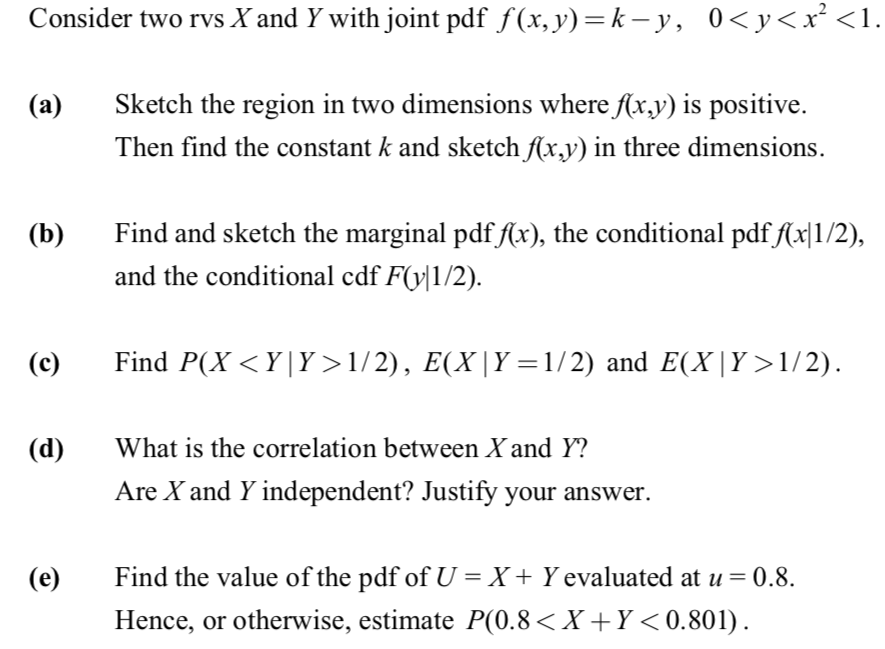0 〈 y 〈 x2く1· Consider two rvs X and Y with joint pdf f(x,y) = k-y, (a) Sketch the region in two dimensions where fx,y) is positive. Then find the constant k and sketch ) in three imesions Then find the constant k and sketch f(r.y) in three dimensions (b) Find and sketch the marginal pdf fx), the conditional pdf(x1/2) and the conditional cdf FO11/2). Find P(X〈Y! Y〉 1/2), E(XİY=1/2) and E(XIY〉l/2). (c) What is the correlation between X...

• ### 2. The joint pdf of random variables X and Y is given by f(x.y) k if...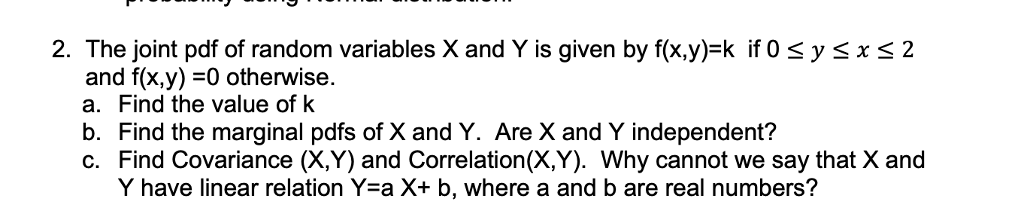2. The joint pdf of random variables X and Y is given by f(x.y) k if 0 sysxs2 and f(x,y)-0 otherwise. a. Find the value of k b. Find the marginal pdfs of X and Y. Are X and Y independent? c. Find Covariance (X,Y) and Correlation(X,Y). Why cannot we say that X and Y have linear relation Yea X+ b, where a and b are real numbers?

• ### The joint pdf of random variables X and Y is given by f(x.y)-k if 0 s...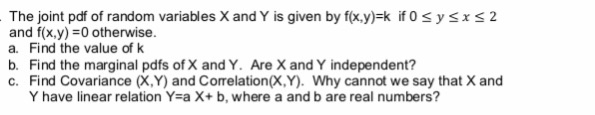The joint pdf of random variables X and Y is given by f(x.y)-k if 0 s y sx s 2 and f(x,y) =0 otherwise. a. Find the value of k b. Find the marginal pdfs of X and Y. Are X and Y independent? c. Find Covariance (X,Y) and Correlation(X,Y). Why cannot we say that X and Y have linear relation Y-a X+ b, where a and b are real numbers?

• ### Suppose the joint pdf of random variables X and Y is f(x,y) = c/x, 0 <...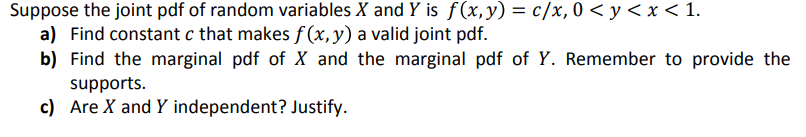Suppose the joint pdf of random variables X and Y is f(x,y) = c/x, 0 < y < x < 1. a) Find constant c that makes f (x, y) a valid joint pdf. b) Find the marginal pdf of X and the marginal pdf of Y. Remember to provide the supports c) Are X and Y independent? Justify

• ### 2. Suppose that the continuous random variable X has the pdf f(x) = cx3:0 < x...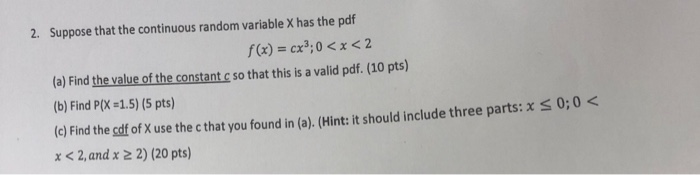2. Suppose that the continuous random variable X has the pdf f(x) = cx3:0 < x < 2 (a) Find the value of the constant c so that this is a valid pdf. (10 pts) (b) Find P(X -1.5) (5 pts) (c) Find the edf of X use the c that you found in (a). (Hint: it should include three parts: x x < 2, and:2 2) (20 pts) 0,0 <

• ### , , Yn is a random sample from a distribution with pdf f,0% θ)-22, 3. (20 points) If Y., Y2, 0 Syse, a. find cÝ, where c is a constant, that is an unbiased estimator of θ; and b. show that the varian...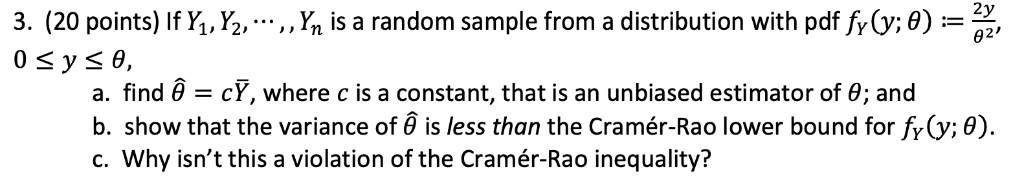, , Yn is a random sample from a distribution with pdf f,0% θ)-22, 3. (20 points) If Y., Y2, 0 Syse, a. find cÝ, where c is a constant, that is an unbiased estimator of θ; and b. show that the variance of is less than the Cramér-Rao lower bound for fr (y; 0) c. Why isn't this a violation of the Cramér-Rao inequality? , , Yn is a random sample from a distribution with pdf f,0% θ)-22, 3....

• ### 1. Determine the constant c such that the given function is a valid joint PDF for...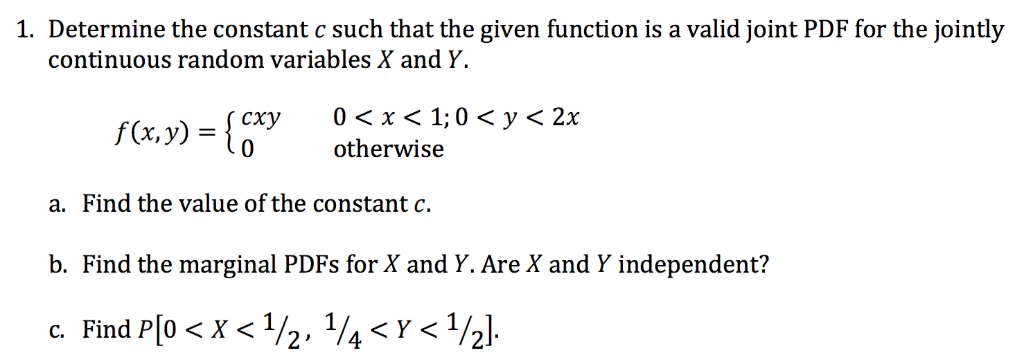1. Determine the constant c such that the given function is a valid joint PDF for the jointly continuous random variables X and Y. f(x,y) ={cry otherwise 0 < y < 2x a. Find the value of the constant c. b. Find the marginal PDFs for X and Y. Are X and Y independent?

• ### Problem 5 20 marks total 0 < y < x2 < Consider two rvs X and Y with joint pdf f(x,y) = k-y, Sketch the region in two dimensions whereAx.y is positive. Then find the constant k and sketch /fx...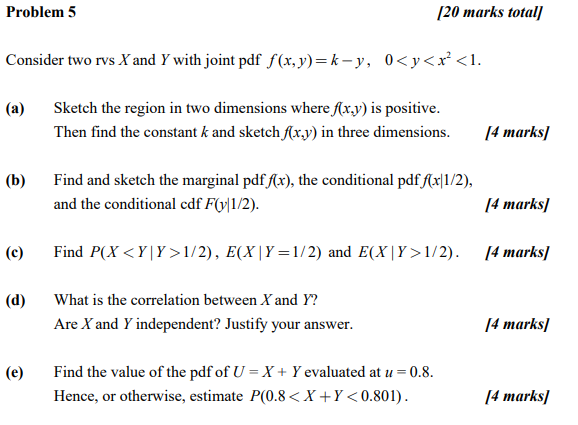Problem 5 20 marks total 0 < y < x2 < Consider two rvs X and Y with joint pdf f(x,y) = k-y, Sketch the region in two dimensions whereAx.y is positive. Then find the constant k and sketch /fx.y) in three dimensions. I4 marksl (a) Find and sketch the marginal pdf/(x), the conditional pdf ffr11/2), and the conditional cdf F11/2) (b) 4 marks] Find P(XcYlY> 1/2), E(XIY=1/2) and E(XlY>1/ 2). /4 marks/ (c) (d) What is the correlation between...

• ### X and Y are jointly uniformly distributed and their joint PDF is given by: fX,Y(x,y) = {k , 0<=x<=4, 0 <=y <= 8 0 , otherwise } a.) find the value of k that makes the joint PDF valid b.) c...

X and Y are jointly uniformly distributed and their joint PDF is given by: fX,Y(x,y) = {k , 0<=x<=4, 0 <=y <= 8 0 , otherwise } a.) find the value of k that makes the joint PDF valid b.) compute the probability P[(X-2)^2 + (Y-2)^2 < 4] c.) compute the probability P[Y > 0.5X + 5]

• ### Let Y = g(x)= x - 3, where the PDF is f(0) { 1 21 0...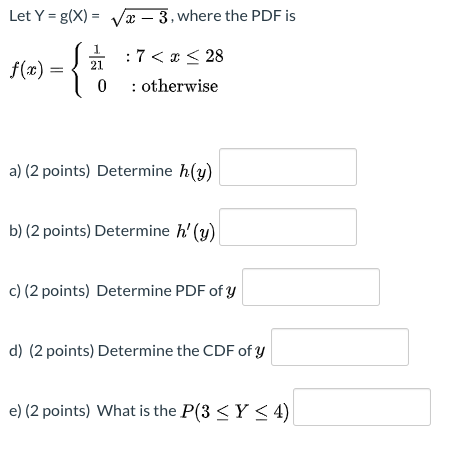Let Y = g(x)= x - 3, where the PDF is f(0) { 1 21 0 : 7 < < 28 : otherwise a) (2 points) Determine h(y) b) (2 points) Determine h' (y) c) (2 points) Determine PDF of y d) (2 points) Determine the CDF of y e) (2 points) What is the P(3<Y < 4)